# Which of the following reactions have a positive ?Srxn? Check all that apply

Which of the following reactions have a positive ?Srxn? Check all that apply.
2A(g) + B(s) --> 3C(g) 2A(g) + B(g) --> C(g) A(g) + B(g) -->C(g) 2A(g) + 2B(g) -->5C(g)

Concepts and reason
Entropy:
The entropy S describes the randomness and disorders of molecules, depending on the number of various arrangements possible for the molecules, in a given system of reaction.

Fundamentals
As the randomness of the molecules increases, the entropy also increases and gives a positive sign.

Conditions for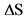to be positive:

1. Entropy increases as the phase changes from solid to liquid, liquid to gas or solid to gas.
2. If the change in number of moles is positive, then the entropy is also positive.

The given reaction is as follows: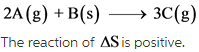Explanation: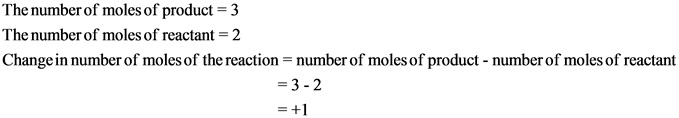The change in number of moles is positive. Hence, the randomness of the molecules increases, which results in giving positive entropy.

The given reaction is as follows:The change in number of moles is negative. Hence, the randomness of the molecules decreases, which results in giving negative entropy.

The given reaction is as follows: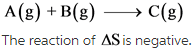Explanation: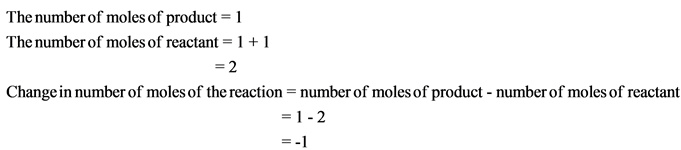The change in number of moles is negative. Hence, the randomness of the molecules decreases, which results in giving negative entropy.

The given reaction is as follows: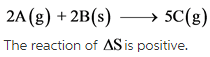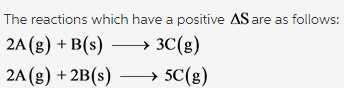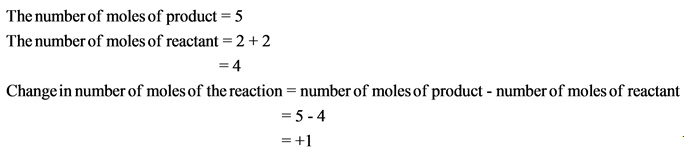The change in number of moles is positive. Hence, the randomness of the molecules increases, which results in giving positive entropy.